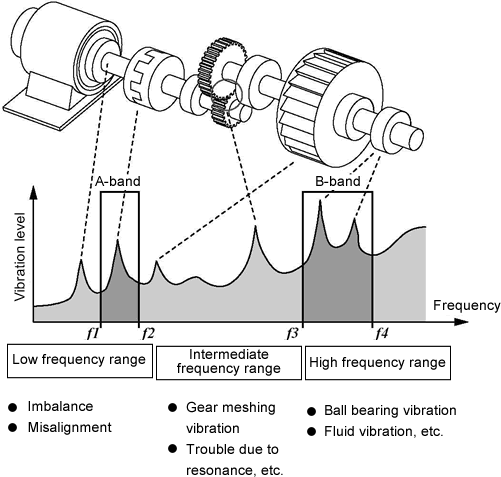Vibration Comparator FAQHow to decide the comparator setup valueWhen you setup the comparator value for the first time;

The frequency that should be remarked is different which should be noticed are different, depending on the measurement object such as crack of the bearing, the core defection of the shaft or imbalance.

1. For the core defection, inclination or imbalance of the shaft, the abnormal vibration appears when the frequency is corresponding to the [shaft rotation speed].

2. For the gear tooth, the abnormal vibration appears when the frequency is corresponding to the [shaft rotation speed × gear tooth number].

3. For the crack of the bearing, the abnormal vibration appears when the frequency is high more than several kHz. So setup the high pass filter at 1 kHz. (We have the VC-3100 Vibration Comparator which is exclusive for the judgment of the bearing crack.)

Set the appropriate band pass frequency depending on the each frequency to be remarked.

Measure the effective value or the peak value of the normal and abnormal vibration, and estimate the judgment level. If the abnormal data cannot be obtained, use the normal value as a reference. For example, the value can be setup as a little higher than the maximum value of the normal value or +3σ (σ: standard deviation). When it is judged as the abnormal data, please reconsider if the comparison value is correct or not by referring the measurement data.

Moreover, if you have FFT analyzer, you can find out which frequency band has a possibility of the abnormality occurrence to setup the band pass finely.

For the VC-2100, two types of band passes (frequency band limit) can be setup individually and the comparison judgment is outputted. The following figure shows the example when the A-band is setup as f1 to f2 and the B-band is setup as f3 to f4.Revised:2002/07/15